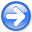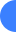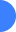Correlation-based wave-equation migration velocity analysisNext: Introduction Up: Reproducible Documents

# Correlation-based wave-equation migration velocity analysis

Ali Almomin

### Abstract:

Wave-equation migration velocity analysis (WEMVA) is a family of techniques that aim to improve the subsurface velocity model by minimizing the residual in the image space. Since the true image is unknown, measuring the residual in the image space is a challenge for WEMVA techniques. In this paper, I present a new method of measuring the image perturbation that is based on the cross-correlation of the observed image with a reference image in reflection angle gathers. I derive the gradient of this technique and show some synthetic examples that compare it to the optimum WEMVA gradient. I then modify the gradient in order to handle multiple events and show that it becomes immune to the problem of cycle skipping. I finally show a synthetic example of the modified gradient and compare it to the optimum gradient.

2011-05-24